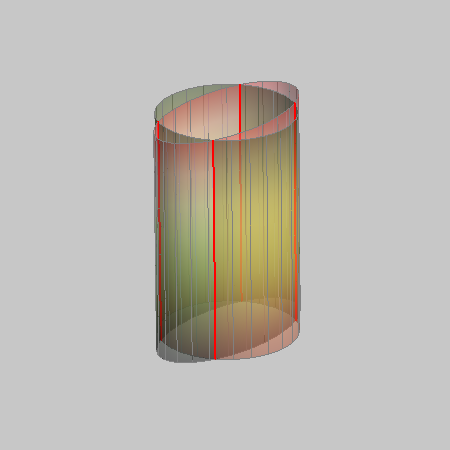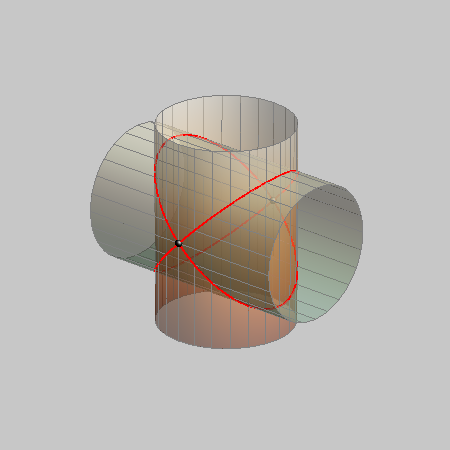### A general principle for the construction of points of the intersection of two cylinders

 We choose a system of planes that are parallel with both axes of the two cylinders. These planes form a pencil of parallel planes. Each plane from that pencil intersects each cylinder in two parallel generatrices. The four intersection points of these generatrices are points of the intersection curve. right-click $$\rightarrow$$ PLAY

### Examples of the intersection of two cylinders

#### Proper curves of the 4th order

There are three types of intersection curves:
• a one-branch curve, which occurs when one cylinder passes only partially through the other.
This type of intersection is called partial intersection.
• a two-branch curve, which occurs when one cylinder passes completely through the other.
This type of intersection is called complete intersection.
• a curve of the 4th order with one double points, which occurs when the cylinders have a common tangent plane.PARTIAL INTERSECTION- a one-branch curve COMPLETE INTERSECTION- a two-branch curve an intersection curve with ONE DOUBLE POINT
right-click $$\rightarrow$$ PLAY

#### Degenerated curves of the 4th order

If two cylinders have parallel axes or parallel generatrices, then they have a common vertex. Such two cylinders intersect at four generatrices that can be real and different, real and coincide or pairs of imaginary lines.
Two cylinders of revolution can not have more than two common real generatrices.

Other possible case of degeneration of an intersection curve of two cylinders is two conics. This case appears when the axes of the cylinders are not parallel and the two cylinders have two common tangent planes.
If the cylinders are the cylinders of revolution, this case appears when their axes intersect and the base circles have the same diameter. The intersection curve in that case is degenerated into two ellipses.four linestwo conics

Created by Sonja Gorjanc, translated by Helena Halas and Iva Kodrnja - 3DGeomTeh - Developing project of the University of Zagreb